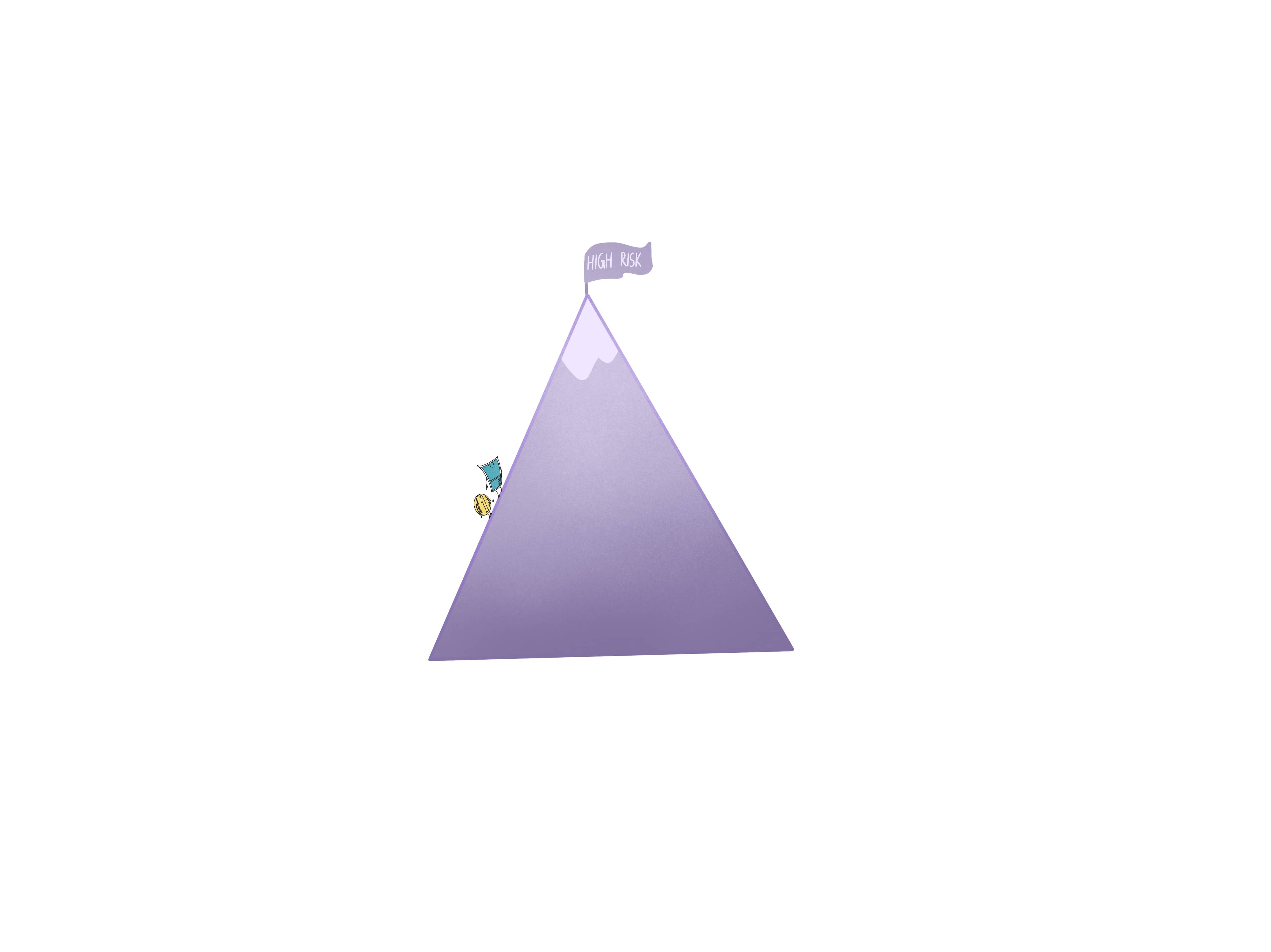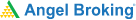# The greek of price movement - DeltaI took a 14-day trip to Goa and it became my most treasured travel experience. On my way back home, I was wondering what made this trip stand out given the fact that I had been to Goa thrice before. Was it the long duration that allowed me to relax and explore? Was it the wonderful beaches I discovered or was it my hotel that offered me the utmost luxury? After giving this a thought, I realised that it was the perfect blend of these aspects that made my trip to Goa so memorable.

My contemplations made me realise the stark similarity between my trip and… options trade. Sounds strange, right?! Well, let me walk you through it. Just like the Goan trip of my dreams, there are various forces in an options trade that come together to make it successful. These forces that work together in making an option trade successful are called Option Greeks. The Option Greeks influence the premium to increase and decrease and at the same time, they also influence each other.

## What are Option Greeks?

There are four Option Greeks:

1. Delta: It measures the rate of change of an option premium.
2. Gamma: The rate of change of Delta is called Gamma.
3. Vega: The rate of change of premium based on the change in volatility is called Vega.
4. Theta: It measures the impact on premium based on time left for expiry.

Let's simplify this with my trip to Goa. South Goa and North Goa are two independent forces. Think of South Goa and North Goa as Option Greeks. These two parts of Goa can independently influence the experience you will have in Goa. If you travel to only one part of Goa, there’s no doubt that it will make for a good trip. However, if you travel to both North and South Goa, then the beauty of one will surely overshadow that of the other.

Now let’s think of this in terms of Option Premium and Option Greeks, and see how the natural demand-supply situation of the markets influence each other. All the factors work independently, and yet have the capability to influence each other. In the Option Premium, the final outcome of this mixture can be assessed. As an options trader, you need to develop a sense for how all the factors influence each other, before setting up an option trade.

### Understanding Option Contract

For hedging a portfolio, you will use Option Contract. Option contracts are used to speculate whether an asset price might rise or fall. This helps offset potential unfavourable moves in other investments. Therefore, the options can be converted to shares of underlying assets at a particularly specified price which is called the strike price.

Here are a few things to remember:

1. There is an end date to every option, which is called an expiration date.
2. The value which is associated with the option is called the premium.
3. The fluctuation in price is based on option pricing models like Black-Scholes.
4. Greeks are usually viewed in conjunction with an option price model to help understand and gauge associated risks.

### Volatility

Volatility is the fluctuation of options premium or market value leading up to the expiration. There can be many reasons which can cause the fluctuation in price. The reasons can be financial conditions of the company, economic conditions, geopolitical risks and the move in overall markets.

Implied volatility represents the market's view of the likelihood that an asset's price will change. Implied volatility is used to anticipate the future of the option's price. If volatility is expected to increase ie. if implied volatility is rising, the premium for an option will likely increase as well.

### Profitability

A few terms are used to describe if an option is profitable or unprofitable.

1. If the difference between the strike price and the price of underlying stock or asset results is a profit, it is called the intrinsic value
2. When the option’s strike price and the underlying asset's price are equal, it is called an at-the-money option
3. When a profit exists due to the option's strike price being more favorable to the underlying price, it is called an in-the-money option.
4. When the option’s strike price is compared with the underlying price, and there is no profit, it is called an out-of-the-money (OTM) option.
5. When the underlying price is higher than the strike price, it is called a put option.

A number of factors can influence an option’s price and these include the Option Greeks that we learned about earlier in this chapter. The Option Greeks (Delta, Gamma, Vega and Theta) are a set of risk measures that are named after the Greek letters that denote them. This indicates how sensitive an option is to time-value decay, changes in implied volatility, and movements in price. Let’s learn about the first of the Option Greeks -- Delta.

Learning & Earning is now super simple₹ 0 Equity Delivery

No Hidden Charges₹ 20 Per Order For Intraday

FAQ,Currencies & CommoditiesZERO Brokerage*

on ALL SegmentsFREE Margin

Trade Funding

## All About Delta

Delta is a number ranging between 0 and 1 that compares the change in the price of an asset to the change in the price of its derivative.

Delta measures the rate of change of premium for every unit change in the underlying. So a delta of 0.2 indicates that for every 1 point change in the underlying, the premium is likely to change by 0.2 units, or for every 100 point change in the underlying the premium is likely to change by 20 points.

As an example, let's assume Nifty at 8000. The 8000 strike price of calls and puts will have Delta of 0.5. Similarly 8100 CE (OTM) may have a delta of 0.4, 8200 CE (far OTM) may be 0.3, and 7900 CE (ITM) may have a Delta of 0.6. Note how they are changing.

Delta Neutral means keeping Deltas of all the options sold the same with any movement of the stock. For example, if stock moves up, the Delta of the call option will increase. So they will move the position a few points up. Essentially, booking profits in the puts and booking losses in the calls – but maintaining the Deltas of the calls and puts more or less the same. The idea is to profit when expiry nears. The Theta will decrease rapidly on both the calls and puts when expiry nears. Ultimately, both the options will expire worthless.

### Probability of Being Profitable

Delta is used when determining the likelihood of an option being in-the-money at expiration. For example, an out-of-the-money call option with a 0.30 delta has roughly a 30% chance of being in-the-money at expiration, whereas a deep-in-the-money call option with a 0.75 delta has a roughly 75% chance of being in-the-money at expiration.

The assumption is that the prices follow a log-normal distribution, like a coin flip.

This means traders can use delta to measure the risk of a given option or strategy. Higher deltas may be suitable for high-risk, high-reward strategies with low win rates while lower deltas may be ideally suited for low-risk strategies with high win rates.

### Delta and Directional Risk

Delta proves helpful when determining directional risk. Positive deltas are long (buy) market assumptions, negative deltas are short (sell) market assumptions, and neutral deltas are neutral market assumptions.

When a call option is bought, you will aim for a positive delta since the price will increase along with the underlying asset price. You aim for a negative delta when you buy a put option, since the price will decrease if the underlying asset price increases.

Top three things to keep in mind with delta:

1. Delta tends to increase closer to expiration for near or at-the-money options.
2. Delta is further evaluated by gamma, which is a measure of delta's rate of change.
3. Delta can also change in reaction to implied volatility changes.

#### Wrapping up

This is how you can use Options Greeks to measure an option’s position, rewards and risks. To understand the probability of a trade making money, one must be able to determine a variety of risk-exposure measurements.

#### A quick recap

1. The Option Greeks (Delta, Gamma, Vega and Theta) are a set of risk measures that are named after the Greek letters that denote them.
2. Option contracts help hedging a portfolio.
3. Implied volatility or implied vol is used to anticipate the future of the option's price.
4. A few terms used to describe the profitability of an option are intrinsic value, at-the-money, in-the-money, out-of-the-money and put option.
5. Delta is a number ranging between 0 and 1 that compares the change in the price of an asset to the change in the price of its derivative.
6. Delta is used when determining the likelihood of an option being in-the-money at expiration.
7. Delta tends to increase closer to expiration for near or at-the-money options.
8. Delta is further evaluated by gamma, which is a measure of delta's rate of change.
9. Delta can also change in reaction to implied volatility changes.Test Your Knowledge

Take the quiz for this chapter & mark it complete.

Comments (0)

Add Comment

Get Information Mindfulness!

Catch-up With Market

News in 60 Seconds.The perfect starter to begin and stay tuned with your learning journey anytime and anywhere.Ready To Trade? Start withOpen an account